### Analyses in the TWOSAMPLEFREQ Statement

#### Overview of the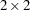Table

Notation:

 Outcome Failure Success Group 1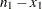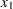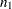2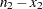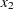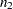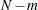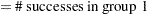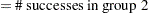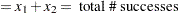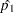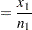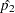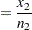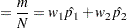The hypotheses are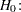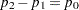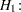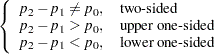where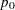is constrained to befor all but the unconditional Pearson chi-square test.

Internal calculations are performed in terms of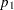,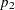, and. An input set consisting of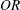,, and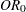is transformed as follows: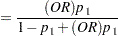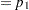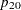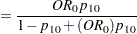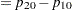An input set consisting of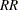,, and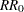is transformed as follows: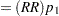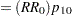Note that the transformation of eitherortois not unique. The chosen parameterization fixes the null valueat the input value of.

#### Pearson Chi-Square Test for Two Proportions (TEST=PCHI)

The usual Pearson chi-square test is unconditional. The test statistic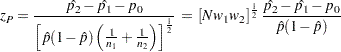is assumed to have a null distribution of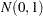.

Sample size for the one-sided cases is given by equation (4) in Fleiss, Tytun, and Ury (1980). One-sided power is computed as suggested by Diegert and Diegert (1981) by inverting the sample size formula. Power for the two-sided case is computed by adding the lower-sided and upper-sided powers each with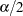, and sample size for the two-sided case is obtained by numerically inverting the power formula. A custom null valuefor the proportion difference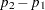is also supported.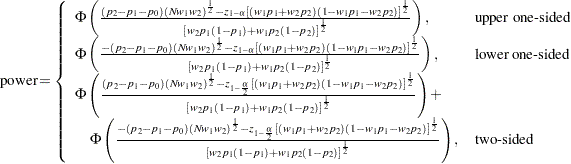For the one-sided cases, a closed-form inversion of the power equation yield an approximate total sample size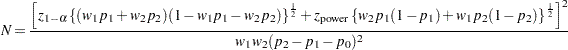For the two-sided case, the solution foris obtained by numerically inverting the power equation.

#### Likelihood Ratio Chi-Square Test for Two Proportions (TEST=LRCHI)

The usual likelihood ratio chi-square test is unconditional. The test statistic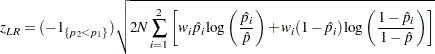is assumed to have a null distribution ofand an alternative distribution of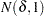, where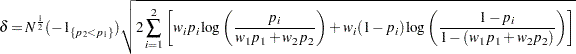The approximate power is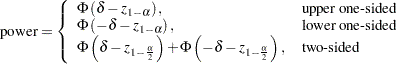For the one-sided cases, a closed-form inversion of the power equation yield an approximate total sample size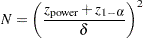For the two-sided case, the solution foris obtained by numerically inverting the power equation.

#### Fisher’s Exact Conditional Test for Two Proportions (Test=FISHER)

Fisher’s exact test is conditional on the observed total number of successes. Power and sample size computations are based on a test with similar power properties, the continuity-adjusted arcsine test. The test statistic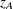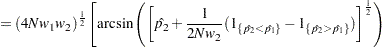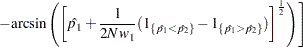is assumed to have a null distribution ofand an alternative distribution of, where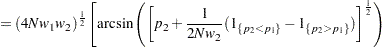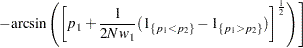The approximate power for the one-sided balanced case is given by Walters (1979) and is easily extended to the unbalanced and two-sided cases: